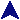PolynomialsSome PVM Examples```#include <stdlib.h>
#include "func.h"
#define MORDER 100

/* Implements a polynomial, given all the coefs.  Max order MORDER */
static double coefs[MORDER];
static int ncoef;
void finit(int argc, char **argv)
{
if(argc > MORDER) {
printf("Too many coefficients: %d provided, max %d.\n",
argc, MORDER);
exit(10);
}
if(argc < 1) {
printf("No coeffiecients specified.\n");
exit(12);
}

int m;
for(m = 0; m < argc; ++m)
coefs[m] = atof(argv[m]);
ncoef = argc;
}

/* Evaluate the poly at x. */
double f(double x)
{
double ret = coefs;
int n;
for(n = 1; n < ncoef; ++n)
ret = ret*x + coefs[n];

return ret;
}
```